# Epsilon-entropy

(diff) ← Older revision | Latest revision (diff) | Newer revision → (diff)
Jump to: navigation, search

of a set in a metric space

The logarithm to the base 2 of the smallest number of points in an-net for this set. In other words, the-entropy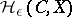of a setlying in a metric spaceis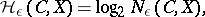where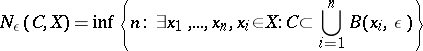andis a ball of radiuswith centre at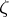. The definition ofwas given by A.N. Kolmogorov in , motivated by ideas and definitions of information theory (cf. Information theory).is also called the relative-entropy; it depends on the spacein which the setis situated, that is, on the metric extension of. The quantity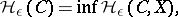where the infimum is taken over all metric extensionsof, is called the absolute-entropy of. It may also be defined directly (Kolmogorov, ): for a metric space,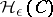is the logarithm to the base 2 of the cardinality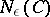of the most economic (by the number of sets)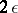-covering of. Here a system of sets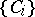is called a-covering ofif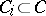,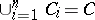and the diameter of each set does not exceed. It has been shown  that the absolute-entropy is the minimum relative-entropy. The quantitiesand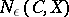are inverse to the widths (cf. Width)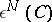and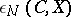. This characterizes the fact that it is possible to recover the elements offrom tables ofelements and to approximate by-point sets.

The investigation of the asymptotic behaviour of the-entropy of various function classes is a special branch of approximation theory.

How to Cite This Entry:
Epsilon-entropy. Encyclopedia of Mathematics. URL: http://encyclopediaofmath.org/index.php?title=Epsilon-entropy&oldid=12568
This article was adapted from an original article by V.M. Tikhomirov (originator), which appeared in Encyclopedia of Mathematics - ISBN 1402006098. See original article